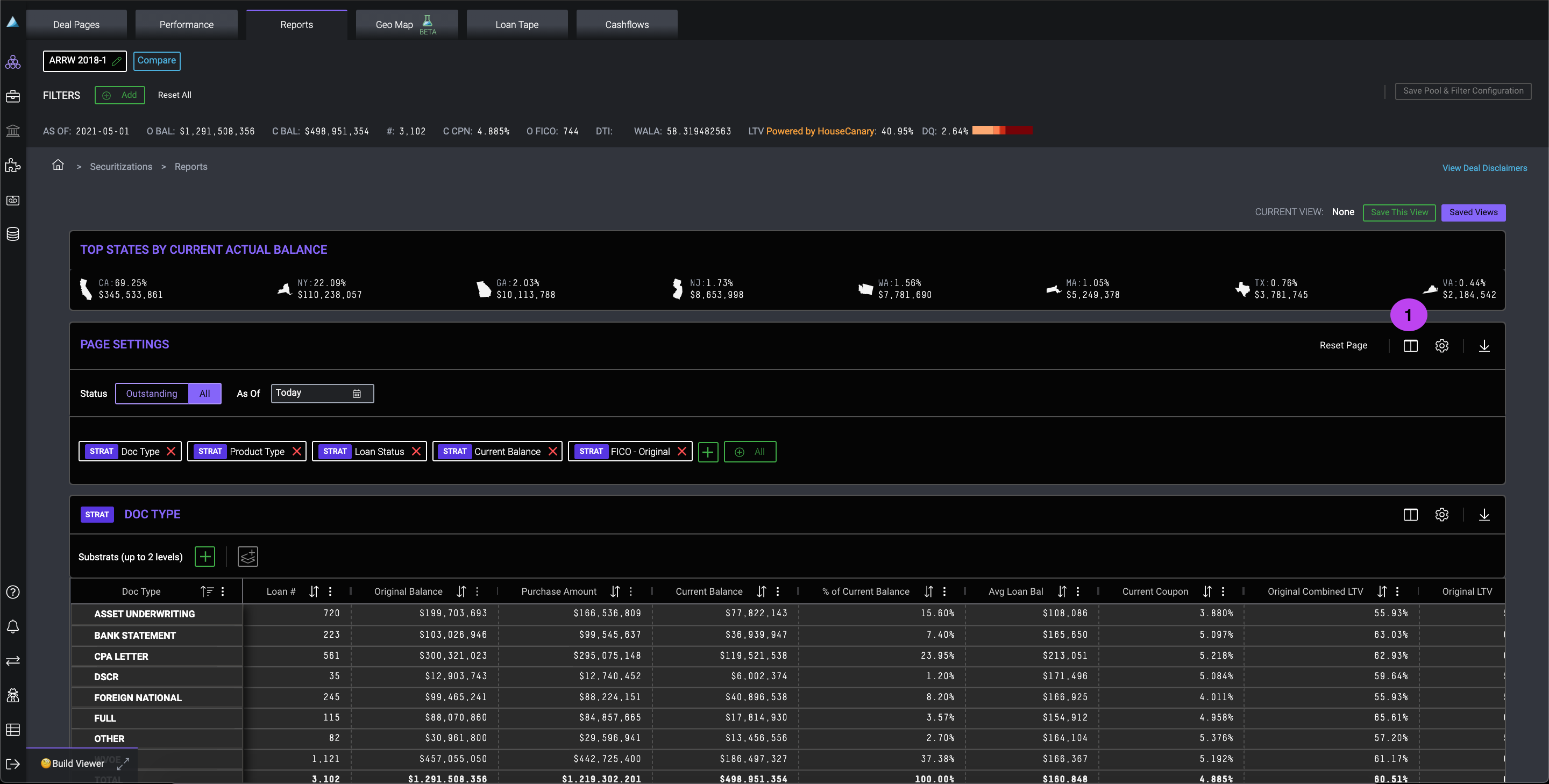Summary

dv01 automatically generates and displays a set of columns based on the fields in your dataset. Users can create and strat by their own columns. Also available on Tape Cracker.

How to:

1. Click "Edit Columns"2. Strat Fields — Within the column editor, you can see all data fields available. To generate a custom column, select “Create New Column”3. Creating a custom column – Name your new field, select your desired function, and complete the remaining required fields. The newly created calculated field will now appear in the data field library. Select the field and hit “save”; it now appears on the Strats page.See it action:

## Supported Aggregation Functions

### Average

• Returns the average of the specified target column.
• Ex.: to create a column to display the average current actual balance of a loan pool, select Average as the function and Current Actual Balance as the target column.

### Count

• Returns the count of loans that meet the specified filter column and filter condition value.
• Ex.: to create a column that counts the loans in California, select Count as the function, Property State as the filter column, and California as the filter condition value.

### Maximum

• Returns the maximum value of the specified target column.
• Ex.: to create a column that returns the maximum loan level Current Actual Balance value, select Maximum as the function and Current Actual Balance as the target column.

### Minimum

• Returns the minimum value of the specified target column.
• Ex.: to create a column that returns the maximum loan level Current Actual Balance value, select Maximum as the function and Current Actual Balance as the target column.

### Non-Zero Weighted Average

• Returns the non-zero weighted average of the specified target column, weighted by the field selected as the Weighted By value. You can only weight columns by numeric columns.
• Ex.: to calculate the non-zero average of Current Actual Balance weighted by original balance, select Non-Zero Weighted Average as the function, Current Actual Balance as the target column, and Original Balance as the Weighted By value.

### Nth Largest Aggregated Percentage

• Returns the target column value with the nth largest total metric (i.e., the nth largest sum of the metric of all loans for a specific target column value), along with the percentage the target column value it represents out of the sum of the entire metric column. This function is currently only available for Tape Cracker.
• Ex.: to display the states with the 3rd largest current actual balances as columns, select Nth Largest Aggregated Percentage as the function, Property State as the target column, Current Actual Balance as the metric, and 3 as the nth value.

### Nth Largest Loan Value

• Returns the target column value with the nth largest individual loan level metric value, along with the value itself. This function is currently only available for Tape Cracker.
• Ex.: to display the property state of the loan with the largest current actual balance value, select Nth Largest Loan Value as the function, Property State as the target column, Current Actual Balance as the metric, and 1 as the nth value.

### Percent of Total

• Returns the percentage of the total target column sum represented by the selected filter column value.
• Ex.: to determine how much of a pool's current actual balance is made up of California loans, select Percent of Total as the function, Current Actual Balance as the target column, Property State as the filter column, and California as the filter column value.

### Sum

• Returns the sum of the target column selected.
• Ex.: to calculate the sum of the Current Actual Balance for each bucket in your strat configuration, select Sum as the function and Current Actual Balance as the target column.

### Weighted Average

• Returns the weighted average of the specified target column weighted by the field selected as the Weighted By value (similar to the Non-Zero Weighted Average function). You can only weight columns by numeric columns.
• Ex.: to calculate the weighted average of Current Actual Balance weighted by original balance, you would select Weighted Average as the function, Current Actual Balance as the target column, and Original Balance as the Weighted By value.